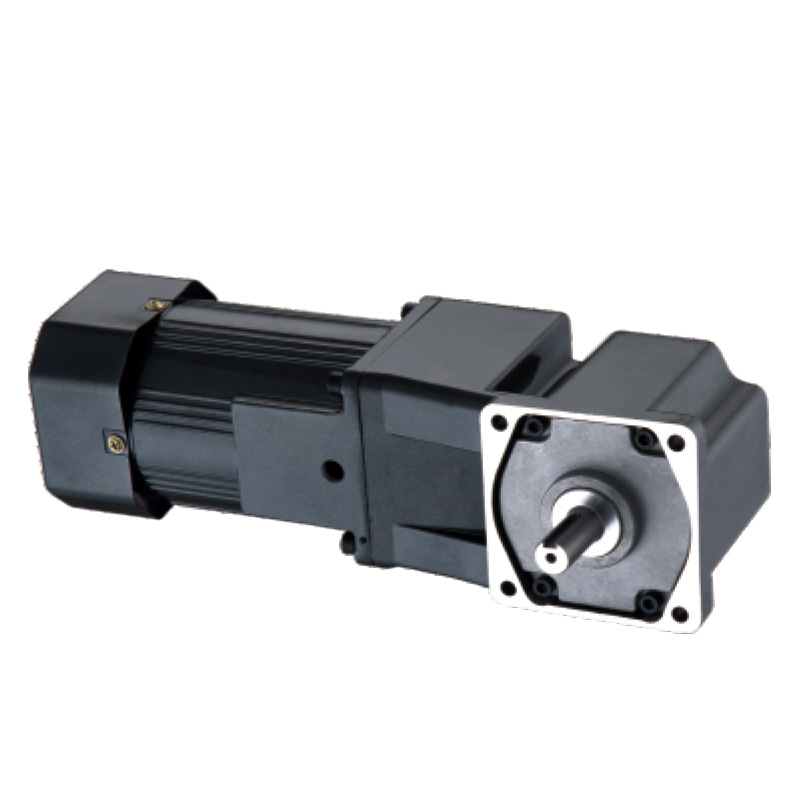# What is the meaning of the number of motor poles, what does it mean?

04/09/2019In the factory, I often hear: "Is the motor a few poles?"

To understand, the first thing to know is what the concept is: The pole refers to the magnetic pole formed by the generator rotor after the rotor coil has passed the excitation current. Simply put, each revolution of the rotor can induce several cycles of different currents in one turn of the coil of the stator. To generate a 50hz potential, different speeds are required.

(50HZ * 60 seconds / minute (ie 3000) divided by the number of turns is the number of turns of the motor per minute) The same is true for the motor, but a reverse process of the generator.

The number of poles reflects the synchronous speed of the motor. The 2-pole synchronous speed is 3000r/min, the 4-pole synchronous speed is 1500r/min, the 6-pole synchronous speed is 1000r/min, and the 8-pole synchronous speed is 750r/min.

Number of poles of the motor:

The number of poles of the motor determines the synchronous speed of the motor

Example: 4-pole motor
4-level motor motor 1 minute synchronous speed = {power frequency (50 Hz) × 60 seconds} ÷ (motor pole number ÷ 2) = 3000 ÷ 2 = 1500 rpm.

It can be understood that the 2 poles are the base number (3000), the 4 poles can only be divided by 2, the 6 poles are divided by 3, and the 8 poles are divided by 4. Instead of saying 2 poles, you need to remove 2 with 3000.

The more pole pairs the motor has, the lower the speed of the motor, but the greater its torque.

Motor selection

When choosing a motor, you need to consider how much starting torque the load requires.

For example, if you start with a load, you need a larger torque than a no-load start. If it is a high-power large load start, also consider the buck start (or star-delta start); as for the problem of matching the speed of the load after determining the number of poles of the motor, consider using different diameter pulleys for transmission or shifting Gears (gearboxes) to match. If the power requirement of the load cannot be reached after passing the belt or gear transmission after determining the number of poles of the motor, then the power consumption of the motor should be considered.

The three-phase AC motor is mainly composed of a stator and a rotor. When a three-phase alternating current is applied to the stator, a rotating magnetic field is generated. The magnetic field always has two poles (also arguably in pairs), namely the N pole (north pole) and the S pole (north pole), also known as a counter pole. When the winding mode of the AC motor stator winding is different, the number of magnetic poles of the generated rotating magnetic field is different. The number of poles directly affects the speed of the motor. Their relationship is: synchronous speed = 60 × frequency / level logarithm. If the synchronous speed of the motor is 1500 rpm, the pole pair number can be calculated according to the above equation, which is a 4-pole motor. The synchronous speed and the number of pole pairs are the basic parameters of the motor and can be found on the nameplate of the motor. Because the pole pair can affect the speed of the motor, you can change the speed of the motor by changing the pole pair of the motor.

For the fluid load of fans and pumps, this type of load has a prominent feature. It is said to be resistant to mutations, which means that such loads have great resistance to sudden changes in the current situation. Although the torque required to drive such load changes is not high, it takes a lot of energy to change the status quo more quickly. It is a bit like boiling water. Small fires can also be boiled. The fire will be great.

The given frequency magnitude and the starting current size are not necessarily related. The magnitude of the starting current depends on the setting of the starting V/F curve and the length of the acceleration time. For fluid loads, the torque required during operation is not constant. Therefore, the use of multiple power curves can make the equipment run more energy-efficient and bring economic benefits to users.

recognition methods:

Look at the speed. For example, the actual synchronous speed of 1430r/min is 1500 rpm, which is calculated by the formula of speed: speed = time (60 seconds) × frequency (50HZ) divided by the number of magnetic poles and one pole pair is 2 poles, so that 3000 ÷ 1500 = Two pole pairs, that is, a 4-pole motor.

Choose the number of poles:

The power of the motor is approximately equal to the pump power divided by the pump efficiency divided by the motor efficiency. The motor efficiency is generally 0.85, your corresponding motor power tells you that it is 5.5KW, 15KW, the speed is 2900, then the motor is 5.5KW-2P and 15KW-2P respectively, the motor speed = (frequency × 60S÷ motor section Number) × 2 .

For example, the choice of pump motor: the selection of the number of poles should be selected according to the rated speed of the pump, 2900r/min for 2 poles, 1450r/min for 4 poles, 970r/min for 6 poles, etc.